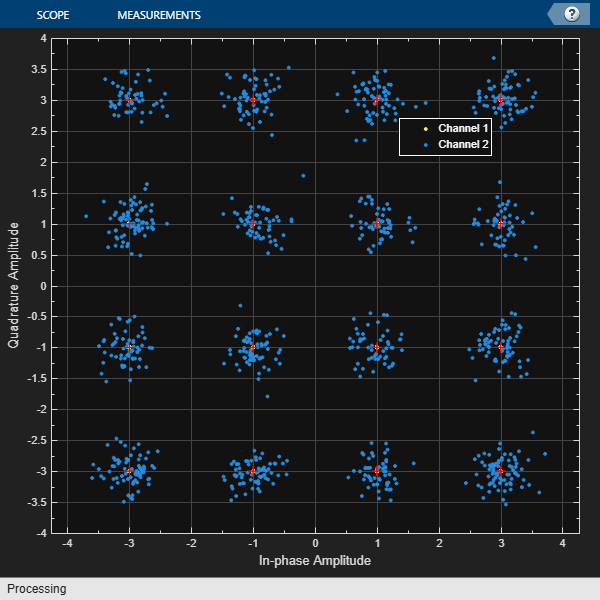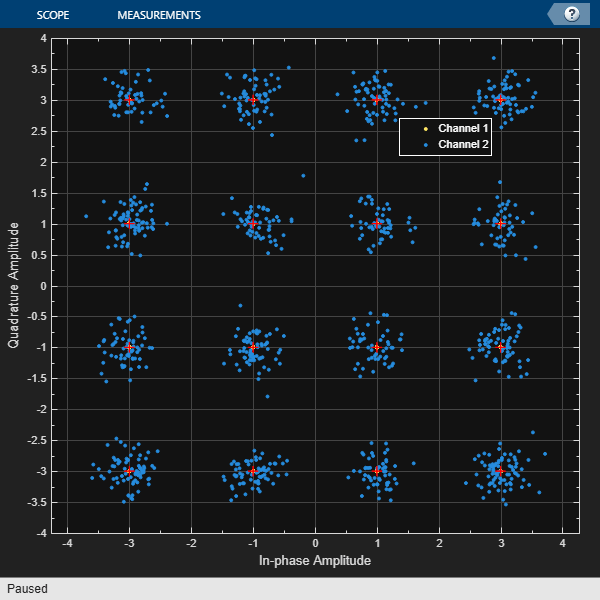Main Content

# hide

Hide scope window

## Syntax

``hide(scope)``

## Description

example

````hide(scope)` hides the window of the System object™ scope.```

## Examples

collapse all

Create a `comm.ConstellationDiagram` object.

`scope = comm.ConstellationDiagram;`

Hide the constellation diagram scope window.

```if(isVisible(scope)) hide(scope) end```

Show the constellation diagram scope window.

```if(~isVisible(scope)) show(scope) end```

Hide the constellation diagram scope window again.

```if(isVisible(scope)) hide(scope) end```

Generate a 16-QAM reference constellation and a signal to display.

```M = 16; xRef = (0:M-1)'; refConst = qammod(xRef,M); signal = randi([0 M-1],1000,1);```

Create a constellation diagram System object™, specifying the constellation reference points and axes limits using name-value pairs.

```scope = comm.ConstellationDiagram('ReferenceConstellation',refConst, ... 'XLimits',[-4 4],'YLimits',[-4 4]);```

Modulate the random data signal using QAM. Add Gaussian white noise to the QAM symbols. Display the QAM symbols and noisy symbols with the constellation diagram object.

```sym = qammod(signal,M); rcv = awgn(sym,20,'measured'); scope([sym rcv]);```Hide the constellation diagram scope window.

```if(isVisible(scope)) hide(scope) end```

Show the constellation diagram scope window.

```if(~isVisible(scope)) show(scope) end```Clear the workspace variables.

```clear scope sym rcv M refConst signal xRef ```

## Input Arguments

collapse all

Scope System object, specified as a `comm.ConstellationDiagram` System object.

Example: ```scope = comm.ConstellationDiagram;```

## See Also

### Objects

Introduced in R2013a

## Support

#### Bridging Wireless Communications Design and Testing with MATLAB

Download white paper